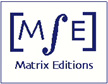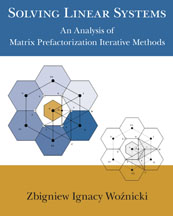Matrix Editions
serious mathematics,
written with the reader in mind

### Solving Linear Systems: An Analysis of Matrix Prefactorization Iterative Methods

#### by Zbigniew Ignacy WoźnickiISBN-13: 9780971576667
554 pages, 8 x 10 inches, hardcover, smythe-sewn binding
More than 230 figures and 140 tables

2009, \$89

Please consider recommending that your university or department librarian order this book. Thank you!

Foreword by Richard Varga

Chapter 0 Introduction to iterative methods and preconditioning

Chapter 1 Numerical linear algebra: background

1.1 Review of matrix theory

Basic matrix operations
Matrix partitionings
Basic concepts of matrix analysis
Vector norms and matrix norms
Computational work

1.2 Eigenvalues and eigenvectors

Relating norms and eigenvalues
Convergence of vector and matrix sequences
Perron-Frobenius theory of nonnegative matrices
Diagonally dominant matrices
Power method

1.3 General linear systems

Direct methods
Iterative refinement

#### Chapter 2 The theory of matrix splitting

2.1 General properties of matrix splittings
2.2 Regular splittings
2.3 Nonnegative and weak nonnegative splittings
2.4 Weak and weaker splittings
2.5 Summary

#### Chapter 3 Discretization of partial differential equations

3.1 Finite-difference approximations
3.2 One-dimensional problems

Forward elimination - backward substitution
Backward elimination - forward substitution

3.3 Band matrices

General band systems

3.4 Two-dimensional problems

Rectangular geometry
Triangular geometry
Hexagonal geometry
Reduced systems
Line orderings
Computational molecules
Irregular mesh structures

3.5 Test problems

#### Chapter 4 Standard iterative methods

4.1 General theory of iterative methods

Stopping criteria
Starting vectors

4.2 Point iterative methods

Basic algorithms Consistent orderings
The successive overrelaxation method (SOR)
Determining the optimum relaxation parameter
Experimental examination of SOR convergence
Computational aspects

4.3 Line iterative methods

1-line algorithms
2-line algorithms
3-line algorithms

4.4 Results of numerical experiments

#### Chapter 5 Explicit prefactorization methods (AGA)

5.1 Matrix notation

Basic algorithms
AGA algorithms with point modification
AGA algorithms with line modification
Techniques for accelerating convergence

5.2 Implementing prefactorization algorithms in mesh structures

Rectangular geometry
Triangular geometry
Hexagonal geometry

5.3 Results of numerical experiments

#### Chapter 6 Semi-explicit prefactorization with implicit backward sweep

6.1 Matrix notation

Modified line methods

6.2 Implementation in mesh structures

Rectangular geometry
Triangular geometry
Hexagonal geometry

6.3 Numerical experiments

Concluding remarks

#### Chapter 7 Semi-explicit prefactorization with implicit forward sweep (OLA)

7.1 Implementing OLA methods in mesh structures

Rectangular geometry
Triangular geometry
Hexagonal geometry
Successive overrelaxation

7.2 Matrix notation

7.3 Numerical experiments

OLA algorithms: concluding remarks

7.4 Final discussion

#### Chapter 8 Advances in solving linear control systems

8.1 Sylvester equations

The SOR-like method v Numerical experiments
Sylvester equations: conclusion

8.2 Continuous-time algebraic Riccati equations

The SOR-like method
Numerical experiments
Concluding remarks